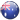# AUS:+61-280-07-5697

The atomic size is very important property of the atoms because it is related to many other chemical and physical properties. In dealing with atomic size, the atom is assumed to be a sphere and its radius determines the size. In general, atomic radius is defined as the distance of closest approach to another identical atom. However, it is not possible to find precisely the radius of the atoms because of the following reasons:

1. Atom is too small to be isolated.

2. The electron cloud of an atom has no well-defined boundary.

It is for this reason that atomic size is expressed in terms of different types of radii. Some of these are being discussed below:

The approximate radii of atoms can be determined by measuring the distance between the atoms in a covalent molecule by X-ray diffraction and other spectroscopic techniques. This radius of an atom is referred to as covalent radius. It may be defined as one-half of the distance between the centres of the nuclei of two similar atoms bonded by a single covalent bond.

In case of homonuclear bonds,

r covalent = 2 [Intern clear distance between two bonded atoms]

For example, the intern clear distance between two hydrogen atoms in H2 molecule is 74 pm (Fig. 6.4). Therefore, the covalent radius of hydrogen atom is 37 pm.Similarly internuclear distance between two chlorine atoms in chlorine molecule is 198 pm. Therefore, covalent radius of chlorine is 19812 = 99 pm.

2 ‘JAN DER WAALS’ RADIUS

Van der Waals ‘ radius may be defined as half of the internuclear distance between two adjacent atoms of the same element belonging to two nearest neighboring molecules of the same substance in solid state.

Covalent radius of the elements is shorter than its van der Waal radius. The formation of covalent bond involves overlapping of atomic orbitals. As a result of this, the internuclear distance between the covalently bonded atoms is less than the internuclear distance between the non-bonded atoms. This has been shown in Fig. 6.5. Thus,Fig. 6.5. Comparison of covalent and van der Waal radii.

3. METALLIC OR CRYSTAL RADIUS

The term is applied for metals only. A metallic lattice is considered to be consisting of Closely packed atoms which are spherical in shape. Metallic radius may be defined as half of the internuclear distance between two adjacent atoms in the metallic lattice. It is measured in angstrom units. Internuclear distances are measured by X-ray studies. The metallic radius of sodium is found to be 186 pm while that of copper is 128 pm.

The metallic radius of an atom is always larger than its covalent radius because metallic bond is weaker than covalent bond. Therefore, two atoms held by covalent bond are closer to each other. For example, the metallic radius of sodium is 186 pm, whereas its covalent radius as determined from its vapours which exist as Na2 molecules is 154 pm. Similarly, metallic radius of potassium is 231 pm whereas covalent radius is 203 pm.

VARIATION OF ATOMIC RADII IN THE PERIODIC TABLE

Atomic radii usually depend upon nuclear charge and number of main energy levels of an atom. The periodic trends in atomic radii have been described as follows:

Table 6.5. Atomic Radii of Elements of Second Period(a) Variation in a Period

In general, the atomic radii decrease with the increase in the atomic number in a period. For example, atomic radii decrease from lithium to fluorine in second period.

The decrease of atomic radii along a period can be explained on the basis of nuclear charge. In moving from left to right across the period, the nuclear charge increases progressively by one unit but the additional electron goes to the same principal shell. As a result, the electron cloud is pulled closer to the nucleus by the increased effective nuclear charge. This causes the decrease of atomic size. The atomic radii of elements of second period are given in Table 6.5.

(b) Variation in a Group

In general, the atomic radii increase from top to bottom within a group of the periodic table. For example, atomic radii increase from lithium to cesium among alkali metals and the similar trend is followed by halogens from fluorine to iodine as shown in Table 6.6.Table 6.6.   Variation of Atomic Radii of Group-1

and Group-17 elements

In moving down a group, the nuclear charge increases with increase in atomic number but at the same time, there is a progressive increase in the principal energy levels. The number of electrons in the outermost shell, however, remains the same. Since, the effect of additional energy level is more  pronounced than the effect of increased nuclear charge, therefore, the effective nuclear charge decreases. Consequently, the distance of the outermost electron from the nucleus increases on going down the group. In other words, the atomic size goes on increasing as we move down a group.

In short,

Atomic radii increase down the group.

Atomic radii decrease across the period